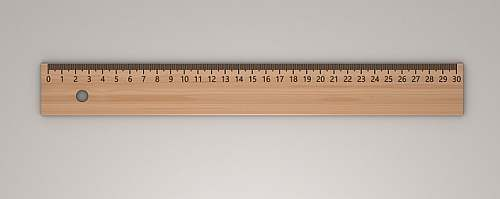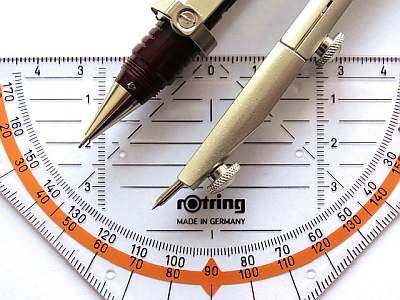# Distance Formula

Instructions: Use this step-by-step Distance Formula calculator, to compute the distance between two points, by typing in the information in the form below:Point 1 (Ex: $$(1, 2)$$, $$(2/3, 1)$$, etc.) =Point 2 (Ex: $$(1, 2)$$, $$(2/3, 1)$$, etc.) =

## Distance Formula Calculator

The distance between two points in the Euclidean plane is one of basic concepts in Geometry. Although, it is not a static or universal concept, as there many potential measures of "distance" in Math.

Indeed, different types of geometry can use different types of distances. And all of those geometries, including the Euclidean geometry, all define distances that are logical and consistent, and hold all the properties that are expected for a distance.

## How do you calculate for distance?

This calculator is based on the distance for the Euclidean geometry. Assume that we have two points $$(x_1, y_1)$$ and $$(x_2, y_2)$$, then the distance formula is computed as follows, using the following formula:

$D = \displaystyle \sqrt{(x_1 - x_2)^2 + (y_1 - y_2)^2}$

This is commonly distance between two points formula, which has the most common interpretation of being the actual physical distance that our senses perceive.

## Why do we calculate distance?

Distance is one of the most basic geometric notions humans have, and the concept of distance has been the foundations of many of the ideas in Geometry, which in turn gives raise to Math as a discipline.

Calculating distances has to do with so many things of a practical, such as how far things are, especially when things are not at close proximity, for which a clear notion of distance plays a crucial role.## Explanation of the Distance formula

The expression above defines how to use the formula for the given two points. What is done is simple: the first component of point 1 and the first component of point 2 are subtracted, and the result is squared.

The same is done for the second point: the second component of point 1 and the second component of point 2 are subtracted, and the result is squared. These two squared values are added up, and you take square root to the result of it. The final number you obtain is the distance

## How do you solve distance problems?

There is no one answer to that question as distance problems can take different forms. Typically, you will be given two points and you will be ask to calculate the distance. That is possibly the easiest type you will get.

But then you can go as hard as you wish. For example, you give to circles (with the corresponding circle equations), and ask which points in the circles are at a certain fixed, given distance $$D$$. Such a problem is definitely harder than the previous one.

Distance formula questions can come in all forms and shapes, and they can be as hard as you can imagine it. Of course in a basic course you will be likely only applying the formula directly.

## What is an example of a distance?

Geometric distances are the most clear examples of distances. For example, if you have a square of side 2 that has its lower-left corner at the origin, and you want to compute the distance between the lowest left corner, and the upper right corner, you compute:

$D = \displaystyle \sqrt{(x_1 - x_2)^2 + (y_1 - y_2)^2} = \displaystyle \sqrt{(0 - 2)^2 + (0 - 2)^2} = \displaystyle \sqrt{2^2 + 2^2} = \displaystyle \sqrt{8} = \displaystyle 2 \sqrt{2}$

There are other examples of distance, with similar interpretation, as the distance you find in Physics. Indeed, those are tightly related but there are plenty of subtleties right there.## In what way this is related to the midpoint formula?

The midpoint formula is tightly related to the distance formula, as the midpoint is a particular point with the special property that the distance from one of the points to it is equal to half of the total distance.

### Examples

Assume that we have two points $$(1, 3)$$ and $$(4, 8)$$, then the distance formula is computed as follows:

$D = \displaystyle \sqrt{(1 - 4)^2 + (3 - 8)^2} = \sqrt{(-3)^2 + (-5)^2} = \sqrt{9 + 25} = \sqrt{34}$

The square root above $$\sqrt 34$$ cannot be simplified any further, so we leave it like that. Sometimes, you will asked to deliver an approximate decimal answer, which in this case would be $$\sqrt 34 \approx 5.8310$$.

### More Examples

How to handle the distance formula with fractions? It is all the same mechanic. Assume that we have two points $$(\frac{1}{2}, \frac{1}{4})$$ and $$(\frac{3}{5}, \frac{3}{4})$$, then the distance formula is computed as follows:

$D = \displaystyle \sqrt{ \left(\frac{1}{2} - \frac{3}{5}\right)^2 + \left(\frac{1}{4} - \frac{3}{4}\right)^2} = \sqrt{\left(-\frac{1}{10}\right)^2 + \left(-\frac{1}{2}\right)^2} = \sqrt{\frac{1}{100} + \frac{1}{4}} = \sqrt{\frac{13}{50}} \approx 5.8310$

### Does the distance have to be in two dimensions?

Not necessarily. Actually we can have two points in a n-dimensional space: $$u = (u_1, u_2, ..., u_n)$$ and $$v = (v_1, v_2, ..., v_n)$$. The distance is now calculated by squaring the differences of all the components, adding them up and taking square root:

$D = \displaystyle \sqrt{(u_1 - v_1)^2 + (u_2 - v_2)^2 + ... + + (u_n - v_n)^2}$### Does distance have anything to do anything with Pythagoras

You bet it does! As your intuition is correctly telling you, the square root of the sum of squares resembles a lot that of the Pythagorean theorem and also what you do when you solve triangles.

This is because we are defining the distance between two points in the Pythagorean way of geometry, as the size of the hypotenuse for a triangle in which the vertices are defined by the points given.

Or alternatively, you can get those two points and compute the midpoint .